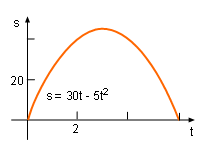Ch 4. The Mean Value Theorem Multimedia Engineering Math Maximum & Minimum Rolle'sTheorem Mean ValueTheorem MonotonicFunctions First DerivativeTest Concavity &Inflection SecondDerivative Test
 Chapter 1. Limits 2. Derivatives I 3. Derivatives II 4. Mean Value 5. Curve Sketching 6. Integrals 7. Inverse Functions 8. Integration Tech. 9. Integrate App. 10. Parametric Eqs. 11. Polar Coord. 12. Series Appendix Basic Math Units Search eBooks Dynamics Fluids Math Mechanics Statics Thermodynamics Author(s): Hengzhong Wen Chean Chin Ngo Meirong Huang Kurt Gramoll ©Kurt GramollMATHEMATICS - CASE STUDY SOLUTIONDisplacement Diagram A ball is kicked directly upward. After the ball reaches its highest altitude, it falls back down to its initial position because of gravity. The altitude function of the ball is s = 30t - 5t2. How high can the ball go up? The for displacement and velocity units are m and m/s, respectively. Since the ball starts and ends at the same vertical location, its displacement function is continuous and differentiable. This satisfies the prerequisite of Rolle's Theorem, and thus a point exists so that the derivative with respect to time, t, is 0.      ds/dt = d(30t -5t2)dt              = d(30t)/dt - d(5t2)/dt              = 30 - 10t As mentioned this must equal zero, ds/dt = 30 - 10t = 0 Since the derivative of displacement with respect to time is velocity, the above equation can be rewritten as      ds/dt = v = 30 - 10t = 0 Therefore, t = 3 Substituting t = 3 into the displacement function      s = 30t - 5t2         = 30(3) - 5(3)2         = 45 m Thus, the maximum altitude of the ball will be 45 m.